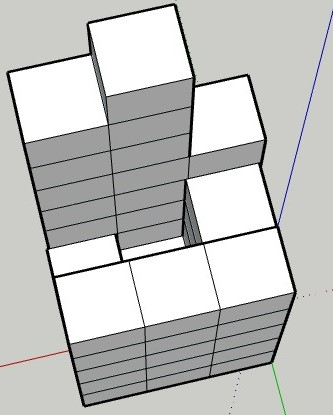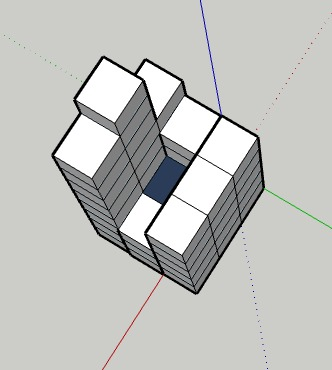Problem title
Difficulty
Avg time to solve

Special Numbers
Moderate
15 mins
Arithmetic Subarrays
Easy
20 mins
Closest Divisors
Moderate
25 mins
Count Number Of Ones
Hard
45 mins
Armstrong Number
Easy
15 mins
Minimum Time To Build Blocks
Easy
15 mins
Self Dividing Numbers
Easy
15 mins
Convert to base '-2'
Moderate
30 mins
NINJA’S KHATA
Moderate
15 mins
Ninja and Cinema Hall
Moderate
30 mins1

# Trapping Rain Water ll

Difficulty: HARD
Avg. time to solve
45 min
Success Rate
55%

Problem Statement

#### Given an M * N matrix, where the value at any cell denotes the height of that cell in a 2-D elevation map. You need to find the volume of water that can be trapped within it.

``````For a matrix =
[ 5, 5, 5 ]
[ 5, 2, 3 ]
[ 6, 9, 8 ]

2-D elevation map will look like this
````````````After the rain, a total of 1 unit volume of water gets trapped, and this 2-D elevation map will look like this
``````##### Input Format:
``````The first line contains a single integer ‘T’ denoting the number of test cases.

The first line of each test case contains two space-separated integers ‘M’ and ‘N’ denoting the number of rows and columns in the matrix.

The next ‘M’ lines contain ‘N’ space-separated integers denoting the height of each cell.
``````
##### Output Format:
``````For each test case, print a single integer denoting the volume of water that can be trapped.

Print the output of each test case in a separated line.
``````
##### Note:
``````You don't need to print anything, it has already been taken care of. Just implement the given function.
``````
##### Constraints:
``````1 <= T <= 10
1 <= M,N <= 100
1 <= matrix[i][j] <= 10^3

Where ‘T’ is the number of test cases, ‘M’ and ‘N’ are the dimensions of the given matrix.
Matrix[i][j] is the height of the cell at the ‘i-th’ row and ‘j-th’ column.

Time Limit: 1 sec.
``````
##### Sample Input 1:
``````2
3 3
5 5 5
5 2 3
6 9 8
5 4
10 11 1 10
11 2 11 10
11 6 8 10
10 11 10 10
11 11 11 11
``````
##### Sample Output 1:
``````1
14
``````
##### Explanation For Sample Input 1:
``````Test Case 1: Only 1 unit volume of water can be trapped between cells with height ‘3’ and ‘2’.

Test Case 2: ‘2’ unit of volume will be stored between matrix [ 2 ][ 2 ] and matrix[ 2 ][ 3 ].
‘4’ unit of volume will be stored between matrix [ 2 ][ 1 ] and matrix[ 2 ][ 3 ].
‘8’ units of volume will be stored between matrix [ 1 ][ 1 ] and matrix[ 2 ][ 3 ].
Therefore a total of ‘14’ unit volume will be trapped.
``````
##### Sample Input 2:
``````2
2 4
3 8 9 2
2 5 7 1
4 4
4 12 8 6
2 1 5 13
2 1 12 7
2 2 10 5
``````
##### Sample Output 2:
``````0
2
``````
##### Explanation For Sample Input 2:
``````Test Case 1: They only have two sides. Thus, to accommodate any water it needs 4 edges. So, no units of water can be trapped between sides.

Test Case 2: ‘1’ unit of volume will be stored between matrix [ 2 ][ 1 ] and matrix[ 2 ][ 2 ].
‘2’ unit of volume will be stored between matrix [ 3 ][ 1 ] and matrix[ 3 ][ 3 ].
Therefore a total of ‘2’ unit volume will be trapped.
``````Console